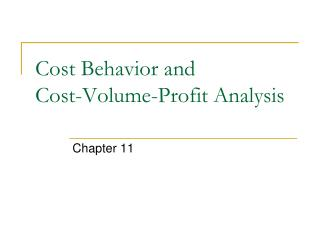# Cost Behavior and Cost-Volume-Profit Analysis - PowerPoint PPT PresentationDownload PresentationCost Behavior and Cost-Volume-Profit Analysis

Cost Behavior and Cost-Volume-Profit AnalysisDownload Presentation## Cost Behavior and Cost-Volume-Profit Analysis

- - - - - - - - - - - - - - - - - - - - - - - - - - - E N D - - - - - - - - - - - - - - - - - - - - - - - - - - -
##### Presentation Transcript

1. Cost Behavior • Cost behavior is the manner in which a cost changes as some related activity changes • An understanding of cost behavior is necessary to plan and control costs • A relevant range is the range over which we are interested in the cost’s behavior

2. Cost Behavior • Variable cost • Cost is constant on a per unit basis, but the total cost varies directly with changes in activity • Materials, fuel, etc. • Fixed cost • Cost is constant in total, but varies inversely with changes in activity • Salaries, property taxes, straight-line depreciation, etc.

3. Cost Behavior • Step cost • Cost which is fixed over small ranges of activity, but varies across wider ranges • Supervision costs, labor costs, etc. • Mixed cost • Cost has both a fixed and a variable component • Utility costs in which you pay a fixed amount to have the service available to you, and a variable charge based on how much you use the utility; rental costs in which you pay a fixed amount per period plus a variable amount based on usage, etc.

4. Cost Behavior • Mixed costs must be separated into their fixed and variable components in order to predict changes in the cost • High-low method • Simple regression • Multiple regression

5. Cost Behavior • High-low method • Compares the points of highest and lowest activities, and their related costs, and calculates the formula for a straight line connecting the two points • Dividing the incremental cost by the incremental units of activity gives the variable cost per unit of activity • The variable cost per unit is substituted into the cost formula to determine the fixed cost • Total cost = fixed cost + variable cost per unit * number of units of activity

6. Cost Behavior

7. Applications of Cost Behavior Concepts • Contribution margin • Excess of sales over variable costs • Contribution is the incremental amount of each sale that is available to cover fixed costs and provide a profit • Knowing the contribution margin allows us to predict changes in net income that will result from a change in sales volume

8. Applications of Cost Behavior Concepts

9. Applications of Cost Behavior Concepts • Contribution margin percentage • Proportion of each sales dollar that is available to cover fixed costs and provide a profit • If sales increase by \$100,000, profit will increase by \$40,000 (\$100,000 * 40%) • Contribution margin per unit • Dollar amount that each unit contributes toward covering fixed costs and providing a profit • If 50 more units are sold, profit will increase by \$20,000 (50 units * \$400)

10. Applications of Cost Behavior Concepts • Breakeven point • Volume of sales needed to earn no profit or no loss • Revenues = total costs Fixed cost + target profit Contribution margin per unit = number of units Fixed cost + target profit Contribution margin percentage = dollars of sales

11. Applications of Cost Behavior Concepts • The breakeven formulas allow us to play “what if” games • What happens if • Sales price is increased (or decreased) • Variable costs are replaced by fixed costs • Volume increases • Additional amounts spent on advertising will increase sales volume • Etc.

12. Applications of Cost Behavior Concepts • Margin of safety • The excess of current sales volume over the breakeven point • In units • Current unit sales – breakeven unit sales • In percentage • (Current sales – breakeven sales) / current sales • The sales figures may be in dollars or units

13. Applications of Cost Behavior Concepts • Operating leverage • Measures the relative mix of fixed and variable costs • Contribution margin / operating income • Can determine the change in operating income that will result from a change in sales by multiplying the % change in sales by the operating leverage • High operating leverage implies high risk, high reward

14. Applications of Cost Behavior Concepts • Breakeven calculations in a multi-product environment • Assume the mix of products sold remains constant • Determine the contribution margin for a “basket” of goods (the normal sales mix) • Calculate the breakeven point as the number of “baskets” needed to break even

15. Applications of Cost Behavior Concepts